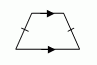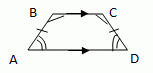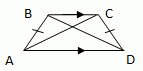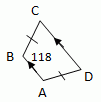Email us to get an instant 20% discount on highly effective K-12 Math & English kwizNET Programs!

#### Online Quiz (WorksheetABCD)

Questions Per Quiz = 2 4 6 8 10

### Geometry3.12 Trapezoid

 Trapezoid Is a quadrilateral with exactly one pair of parallel sides. The parallel sides are the bases A trapezoid has two pairs of base angles. For example in the trapezoid ABCD,�A and �B are one pair of base angles, and �C and �D are the second pair. The non parallel sides are the legs of the trapezoid. Isosceles Trapezoid: If the legs of the trapezoid are congruent then the trapezoid is an Isosceles trapezoid.THEOREMS: If a trapezoid is isosceles, then each pair of base angles is congruent. If trapezoid ABCD is isosceles, then �A @ �D and �B @ �C.If a trapezoid has a pair of congruent base angles, then it is an isosceles trapezoid ABCD is isosceles. If �A @ �D and �B @ �C. then ABCD is isosceles.A trapezoid is isosceles if and only if its diagonals are congruent. Trapezoid ABCD is isosceles if and only if AC @ BD.Midsegment Theorem for Trapezoids: The midsegment of a trapezoid is parallel to each base and its length is one half the sum of the lengths of the bases. If MN is the midsegment of trapezoid ABCD, then MN // AB, MN // DC, and MN = 1/2(AB+CD)Directions: 1) For the trapezoid ABCD shown below find the measures of angle A, angle C and angle D.2) Answer the following questions and write at least 5 examples of your own.
 Q 1: A trapezoid is a quadrilateral with exactly ___ pair of parallel sides.twoonethree Q 2: If the legs of a trapezoid are congruent, then the trapezoid is an ________________.scalene trapezoidisosceles trapezoidequilateral trapezoid Q 3: A trapezoid has two pairs of _____ angles.baseleg Q 4: ABCD is a trapezoid. Using the mid segment theorem find the value of x.Answer: Question 5: This question is available to subscribers only! Question 6: This question is available to subscribers only!